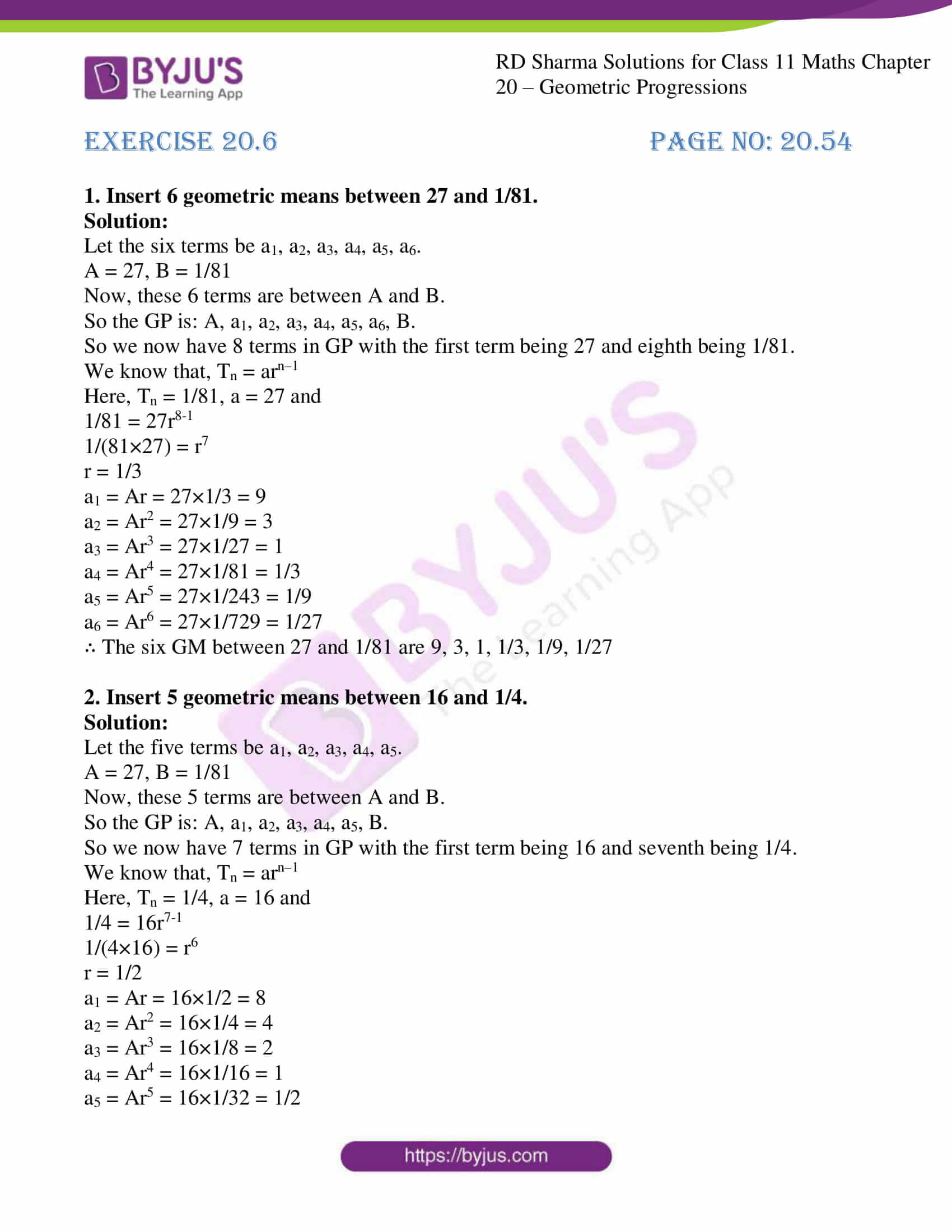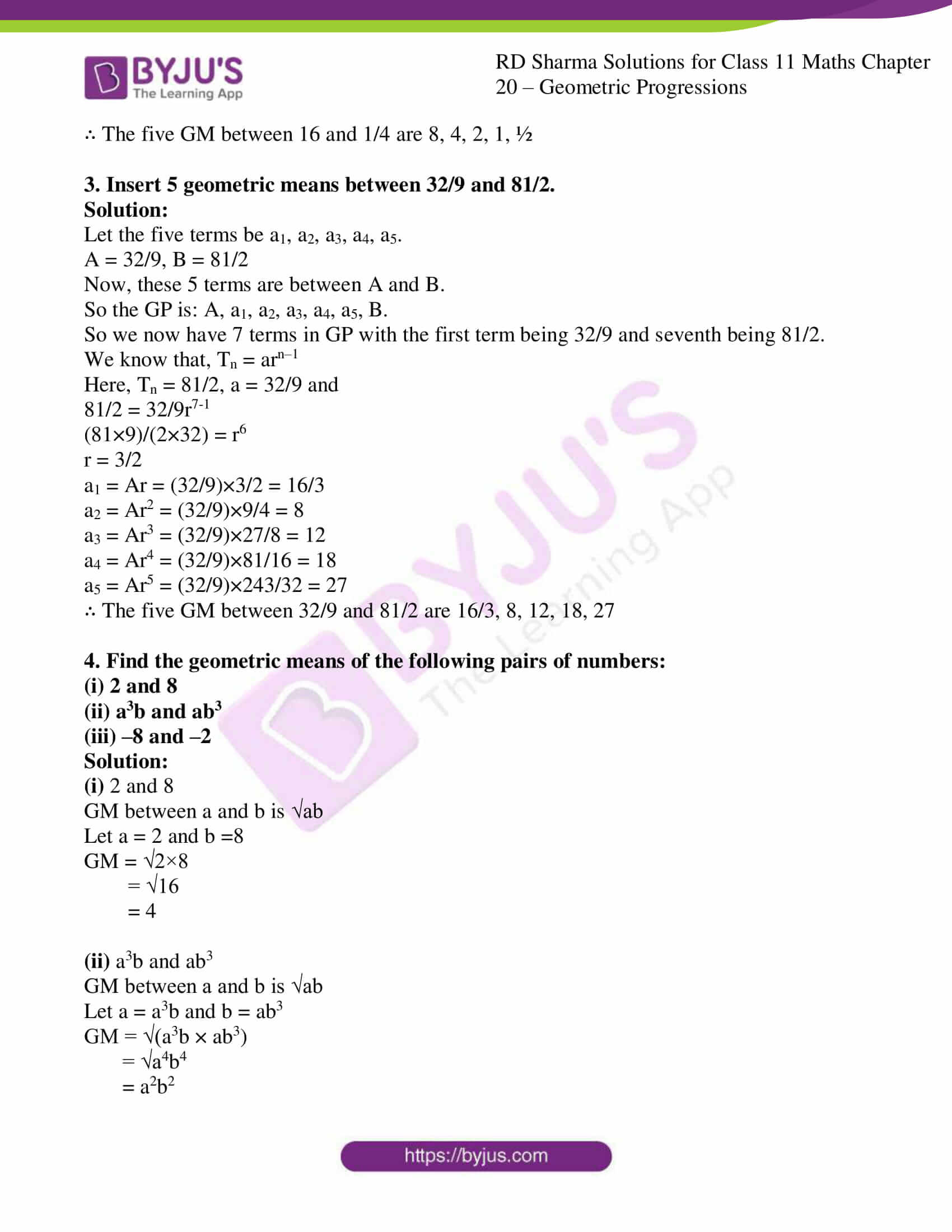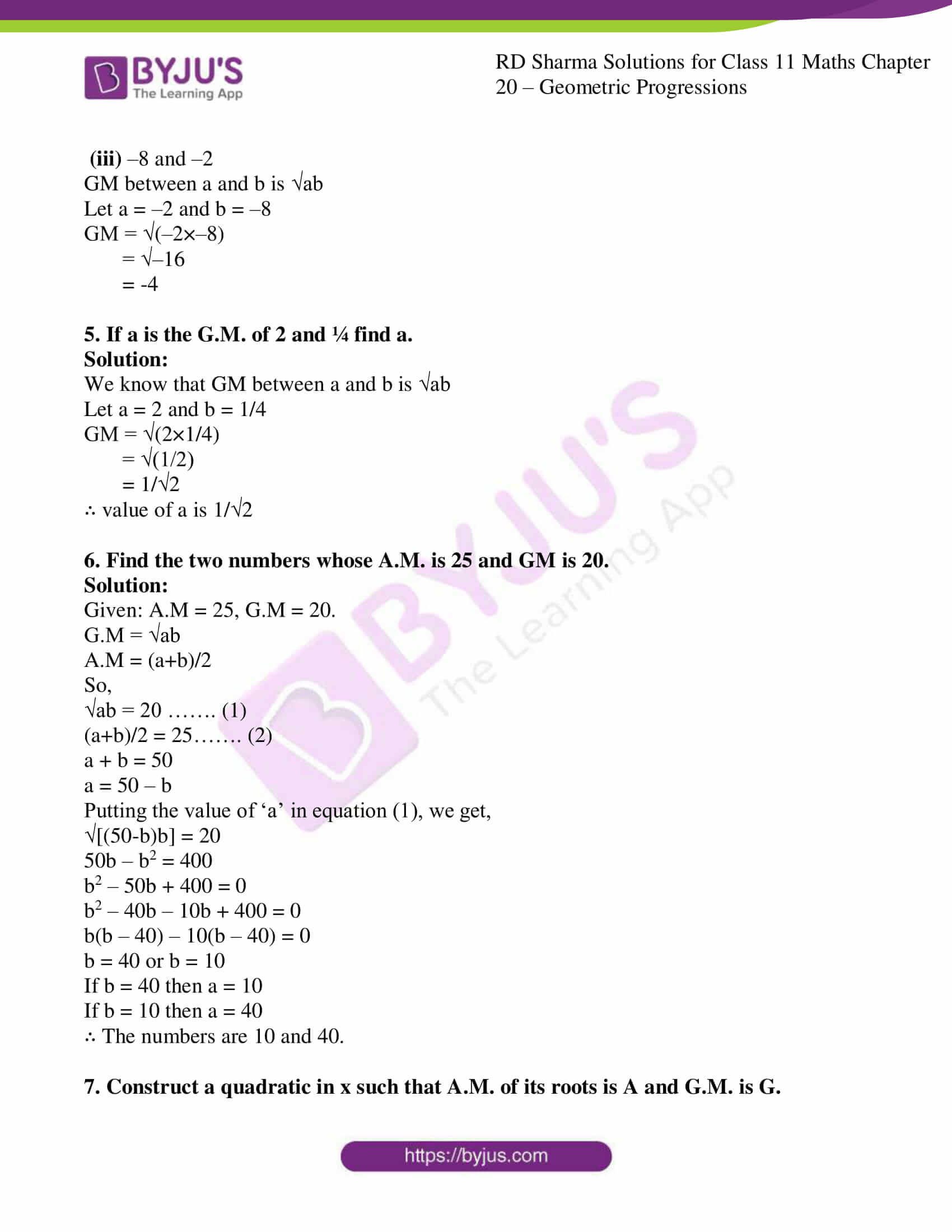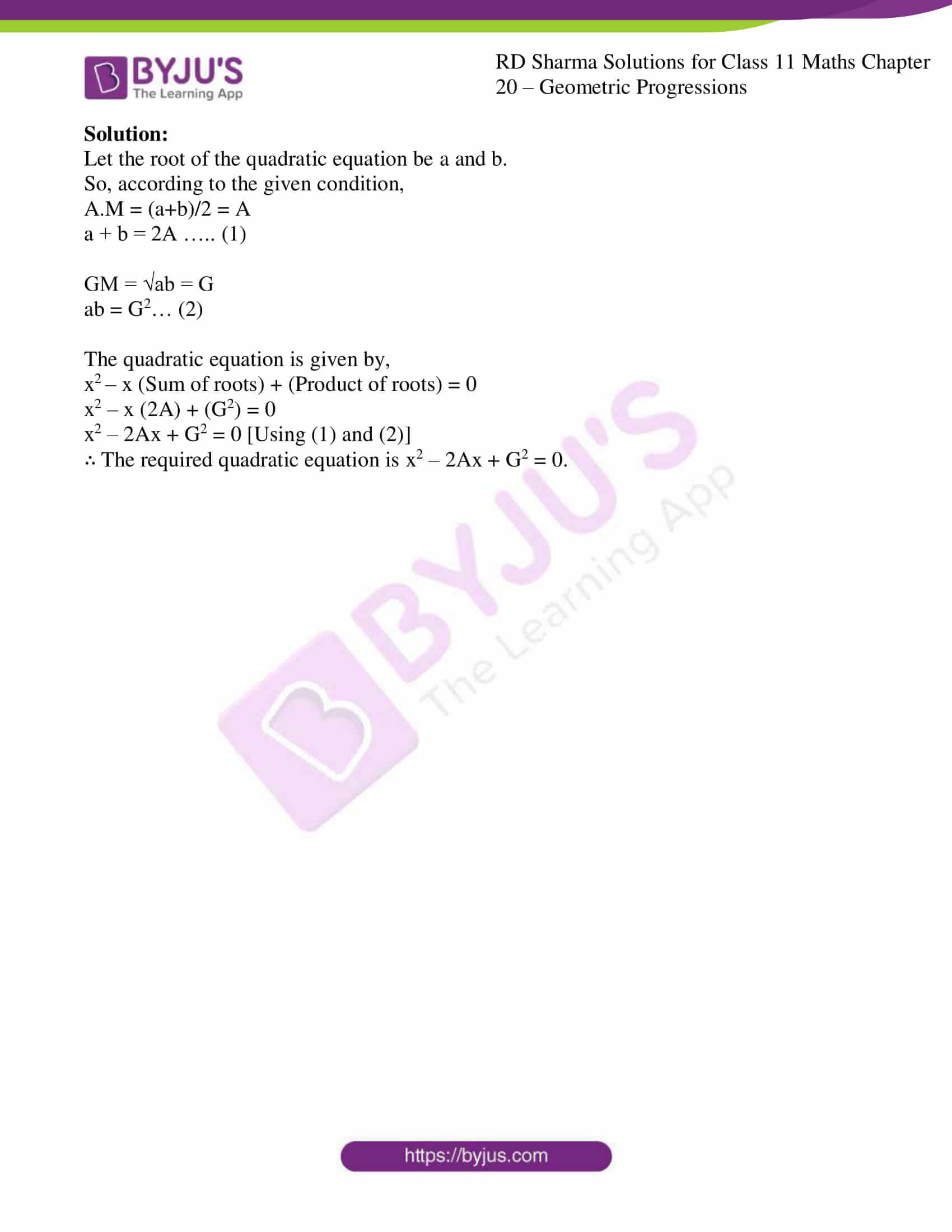# RD Sharma Solutions Class 11 Geometric Progressions Exercise 20.6

This exercise deals with concepts pertaining to insertion of geometric means between two given numbers and we also deal with some important properties of arithmetic and geometric means. Expert tutors have created the RD Sharma Solutions aiming to help students attain the correct methods of solving problems. In order to strengthen problem-solving abilities, students can make use of RD Sharma Class 11 Maths Solutions to achieve their goals. Students can easily download the readily available pdf of RD Sharma Solutions from the links given below and can start practising offline.

## Download the pdf of RD Sharma Solutions for Class 11 Maths Exercise 20.6 Chapter 20 – Geometric Progression### Also, access other exercises of RD Sharma Solutions for Class 11 Maths Chapter 20 – Geometric Progression

Exercise 20.1 Solutions

Exercise 20.2 Solutions

Exercise 20.3 Solutions

Exercise 20.4 Solutions

Exercise 20.5 Solutions

### Access answers to RD Sharma Solutions for Class 11 Maths Exercise 20.6 Chapter 20 – Geometric Progression

1. Insert 6 geometric means between 27 and 1/81.

Solution:

Let the six terms be a1, a2, a3, a4, a5, a6.

A = 27, B = 1/81

Now, these 6 terms are between A and B.

So the GP is: A, a1, a2, a3, a4, a5, a6, B.

So we now have 8 terms in GP with the first term being 27 and eighth being 1/81.

We know that, Tn = arn–1

Here, Tn = 1/81, a = 27 and

1/81 = 27r8-1

1/(81×27) = r7

r = 1/3

a1 = Ar = 27×1/3 = 9

a2 = Ar2 = 27×1/9 = 3

a3 = Ar3 = 27×1/27 = 1

a4 = Ar4 = 27×1/81 = 1/3

a5 = Ar5 = 27×1/243 = 1/9

a6 = Ar6 = 27×1/729 = 1/27

∴ The six GM between 27 and 1/81 are 9, 3, 1, 1/3, 1/9, 1/27

2. Insert 5 geometric means between 16 and 1/4.

Solution:

Let the five terms be a1, a2, a3, a4, a5.

A = 27, B = 1/81

Now, these 5 terms are between A and B.

So the GP is: A, a1, a2, a3, a4, a5, B.

So we now have 7 terms in GP with the first term being 16 and seventh being 1/4.

We know that, Tn = arn–1

Here, Tn = 1/4, a = 16 and

1/4 = 16r7-1

1/(4×16) = r6

r = 1/2

a1 = Ar = 16×1/2 = 8

a2 = Ar2 = 16×1/4 = 4

a3 = Ar3 = 16×1/8 = 2

a4 = Ar4 = 16×1/16 = 1

a5 = Ar5 = 16×1/32 = 1/2

∴ The five GM between 16 and 1/4 are 8, 4, 2, 1, ½

3. Insert 5 geometric means between 32/9 and 81/2.

Solution:

Let the five terms be a1, a2, a3, a4, a5.

A = 32/9, B = 81/2

Now, these 5 terms are between A and B.

So the GP is: A, a1, a2, a3, a4, a5, B.

So we now have 7 terms in GP with the first term being 32/9 and seventh being 81/2.

We know that, Tn = arn–1

Here, Tn = 81/2, a = 32/9 and

81/2 = 32/9r7-1

(81×9)/(2×32) = r6

r = 3/2

a1 = Ar = (32/9)×3/2 = 16/3

a2 = Ar2 = (32/9)×9/4 = 8

a3 = Ar3 = (32/9)×27/8 = 12

a4 = Ar4 = (32/9)×81/16 = 18

a5 = Ar5 = (32/9)×243/32 = 27

∴ The five GM between 32/9 and 81/2 are 16/3, 8, 12, 18, 27

4. Find the geometric means of the following pairs of numbers:
(i) 2 and 8
(ii) a3b and ab3
(iii) –8 and –2

Solution:

(i) 2 and 8

GM between a and b is √ab

Let a = 2 and b =8

GM = √2×8

= √16

= 4

(ii) a3b and ab3

GM between a and b is √ab

Let a = a3b and b = ab3

GM = √(a3b × ab3)

= √a4b4

= a2b2

(iii) –8 and –2

GM between a and b is √ab

Let a = –2 and b = –8

GM = √(–2×–8)

= √–16

= -4

5. If a is the G.M. of 2 and ¼ find a.

Solution:

We know that GM between a and b is √ab

Let a = 2 and b = 1/4

GM = √(2×1/4)

= √(1/2)

= 1/√2

∴ value of a is 1/√2

6. Find the two numbers whose A.M. is 25 and GM is 20.

Solution:

Given: A.M = 25, G.M = 20.

G.M = √ab

A.M = (a+b)/2

So,

√ab = 20 ……. (1)

(a+b)/2 = 25……. (2)

a + b = 50

a = 50 – b

Putting the value of ‘a’ in equation (1), we get,

√[(50-b)b] = 20

50b – b2 = 400

b2 – 50b + 400 = 0

b2 – 40b – 10b + 400 = 0

b(b – 40) – 10(b – 40) = 0

b = 40 or b = 10

If b = 40 then a = 10

If b = 10 then a = 40

∴ The numbers are 10 and 40.

7. Construct a quadratic in x such that A.M. of its roots is A and G.M. is G.

Solution:

Let the root of the quadratic equation be a and b.

So, according to the given condition,

A.M = (a+b)/2 = A

a + b = 2A ….. (1)

GM = √ab = G

ab = G2… (2)

The quadratic equation is given by,

x2 – x (Sum of roots) + (Product of roots) = 0

x2 – x (2A) + (G2) = 0

x2 – 2Ax + G2 = 0 [Using (1) and (2)]

∴ The required quadratic equation is x2 – 2Ax + G2 = 0.